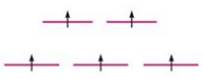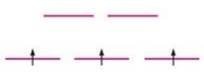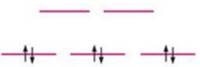# Match the crystal field diagrams given below with the following complex ions. Cr(NH 3 ) 5 Cl 2+ Co(NH 3 ) 4 Br 2 + (assume strong field) Fe(H 2 O) 6 3+ (assume weak field) (a) (b) (c)### Chemistry: An Atoms First Approach

2nd Edition
Steven S. Zumdahl + 1 other
Publisher: Cengage Learning
ISBN: 9781305079243

#### Solutions

Chapter
Section### Chemistry: An Atoms First Approach

2nd Edition
Steven S. Zumdahl + 1 other
Publisher: Cengage Learning
ISBN: 9781305079243
Chapter 20, Problem 49E
Textbook Problem
3 views

## Match the crystal field diagrams given below with the following complex ions. Cr(NH3)5Cl2+ Co(NH3)4Br2+ (assume strong field) Fe(H2O)63+ (assume weak field) (a)(b)(c)(a)

Interpretation Introduction

Interpretation: The given crystal field diagrams are to be matched with the correct complex ions.

Concept introduction: Weak field ligand that has small crystal field splitting energy gives high spin due to presence of maximum unpaired electrons in lower energy t2g orbitals and higher energy orbitals.

Strong field ligand that has large crystal field splitting energy gives low spin due to the presence of maximum paired electrons in lower energy t2g orbitals.

To determine: If the given crystal field diagram corresponds to Cr(NH3)5Cl2+,Co(NH3)4Br2+ ion or Fe(H2O)63+ ion.

### Explanation of Solution

The given crystal field diagram is,

Figure 1

The complex compound is Fe(H2O)63+ (Weak field).

Oxidation state of neutral Water (H2O) is 0.

It is assumed that the oxidation state of metal ion Iron (Fe) is x.

The given complex compound contains six water molecules. Since, the overall charge on the stable compound is +3

(b)

Interpretation Introduction

Interpretation: The given crystal field diagrams are to be matched with the correct complex ions.

Concept introduction: Weak field ligand that has small crystal field splitting energy gives high spin due to presence of maximum unpaired electrons in lower energy t2g orbitals and higher energy orbitals.

Strong field ligand that has large crystal field splitting energy gives low spin due to the presence of maximum paired electrons in lower energy t2g orbitals.

To determine: If the given crystal field diagram corresponds to Cr(NH3)5Cl2+,Co(NH3)4Br2+ ion or Fe(H2O)63+ ion.

(c)

Interpretation Introduction

Interpretation: The given crystal field diagrams are to be matched with the correct complex ions.

Concept introduction: Weak field ligand that has small crystal field splitting energy gives high spin due to presence of maximum unpaired electrons in lower energy t2g orbitals and higher energy orbitals.

Strong field ligand that has large crystal field splitting energy gives low spin due to the presence of maximum paired electrons in lower energy t2g orbitals.

To determine: If the given crystal field diagram corresponds to Cr(NH3)5Cl2+,Co(NH3)4Br2+ ion or Fe(H2O)63+ ion.

### Still sussing out bartleby?

Check out a sample textbook solution.

See a sample solution

#### The Solution to Your Study Problems

Bartleby provides explanations to thousands of textbook problems written by our experts, many with advanced degrees!

Get Started

Find more solutions based on key concepts
This controversy addresses accusations launched against sugars in foods and beverages as causes of health probl...

Nutrition: Concepts and Controversies - Standalone book (MindTap Course List)

Expalin the difference between cytosol and cytoplasm in both prokaryotes and eukaryotes.

Biology: The Unity and Diversity of Life (MindTap Course List)

If three unequal capacitors, initially uncharged, are connected in series across a battery, which of the follow...

Physics for Scientists and Engineers, Technology Update (No access codes included)

What methods of ocean energy extraction have been shown to be practical?

Oceanography: An Invitation To Marine Science, Loose-leaf Versin

What do we call the central part of an atom?

Introductory Chemistry: An Active Learning Approach